# Introduction

The neverhpfilter package consists of 2 functions, 12 economic data sets, Robert Shiller’s U.S. Stock Market and CAPE Ratio data from 1871 through 2019, and a data.frame containing the original filter estimates found on table 2 of Hamilton (2017) <doi:10.3386/w23429>. All data objects are stored as .Rdata files in eXtensible Time Series (xts) format.

One of the first things to know about the neverhpfilter package is that it’s functions accept and output, xts objects.

An xts object is a list consisting of a vector index of some date/time class paired with a matrix object containing data of type numeric. data.table is also heavily used in finance and has efficient date/time indexing capabilities as well. It is useful when working with large data.frame like lists containing vectors of multiple data types of equal length. If using data.table or some other index based time series data object, merging the xts objects created by functions of this package should be fairly easy. Note xts is a dependency listed under the “Suggests” field of data.table DESCRIPTION file.

For more information on xts objects, go here and here.

## yth_glm

The yth_glm function wraps glm and primarily exists to model the output for the yth_filter. On that note, the function API allows one to use the ... to pass any additional arguments to glm.

The yth_filter returns an object of class glm, so one can use all generic methods associated with glm objects. Here is an example of passing the results of a yth_glm model to the plot function, which outputs the standard plot diagnostics associated with the method.

library(neverhpfilter)

data(GDPC1)

log_RGDP <- 100*log(GDPC1)

gdp_model <- yth_glm(log_RGDP["1960/"], h = 8, p = 4)

plot(gdp_model)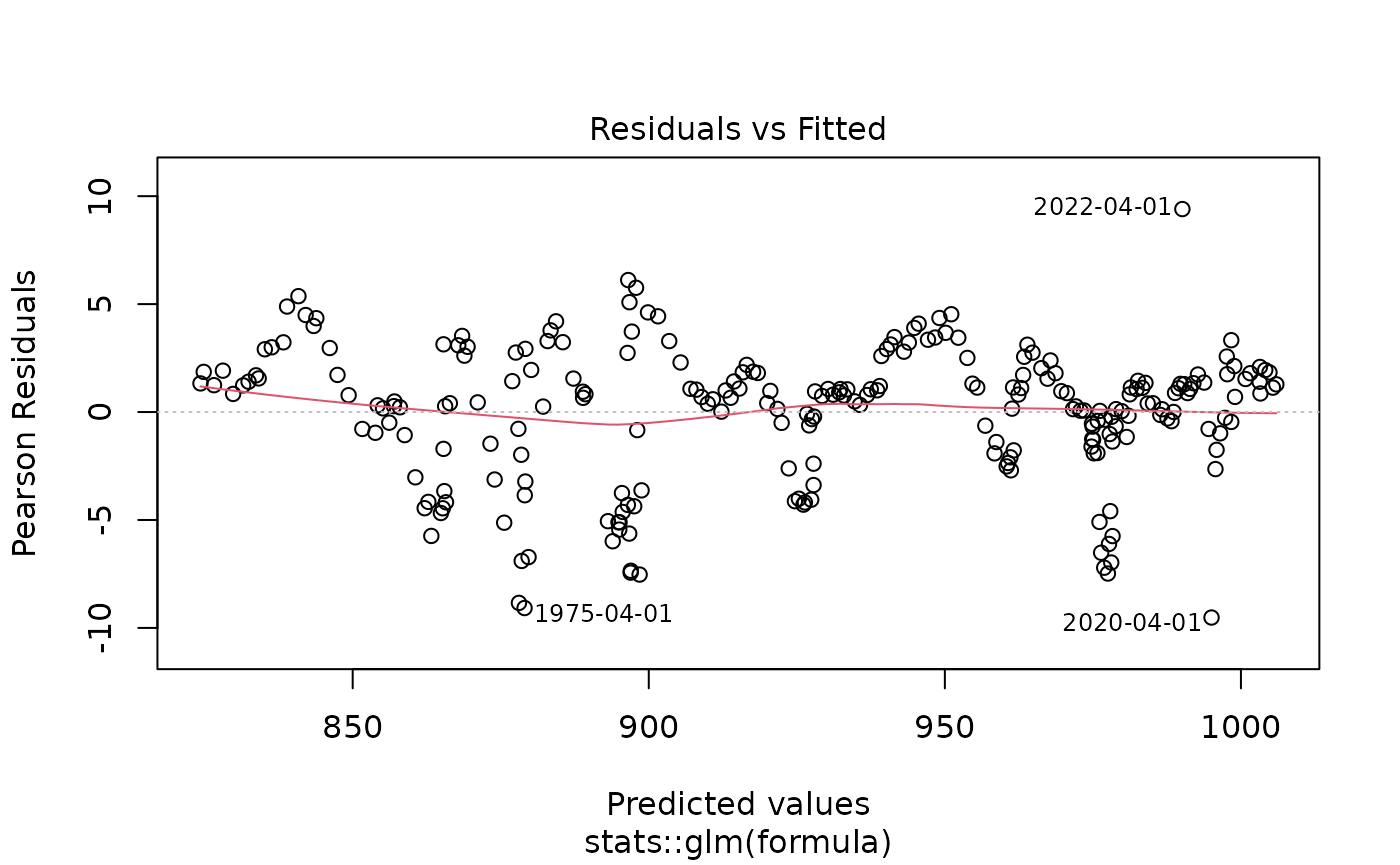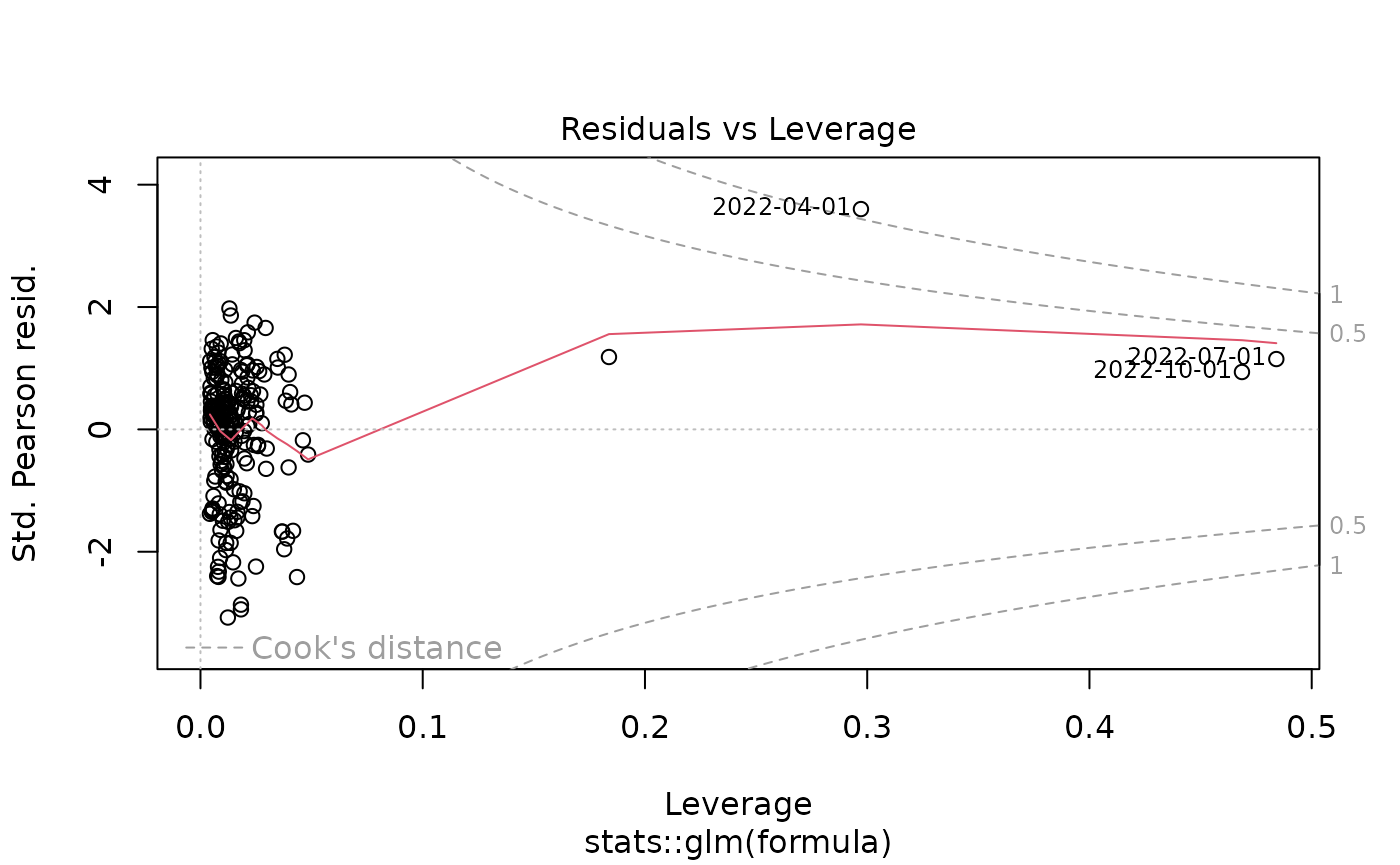## yth_filtered

This is the main function of the package. It both accepts and outputs xts objects. The resulting output contains various series discussed in Hamilton (2017). These are a user defined combination of the original, trend, cycle, and random walk series. See documentation and the original paper for further details.

gdp_filtered <- yth_filter(log_RGDP, h = 8, p = 4)

tail(gdp_filtered, 16)
##            GDPC1 GDPC1.trend  GDPC1.cycle GDPC1.random
## 2017 Q2 980.1125    980.5180  -0.40546176    3.5587871
## 2017 Q3 980.8387    980.7418   0.09688729    3.9230290
## 2017 Q4 981.7899    980.9952   0.79471218    4.7139347
## 2018 Q1 982.7172    981.7481   0.96918064    5.0764851
## 2018 Q2 983.3836    982.0011   1.38255033    5.4314549
## 2018 Q3 983.9075    982.4911   1.41636940    5.4123565
## 2018 Q4 984.2352    983.1578   1.07740709    5.1128136
## 2019 Q1 984.9578    983.6123   1.34540590    5.2712447
## 2019 Q2 985.3278    983.9876   1.34017177    5.2152403
## 2019 Q3 985.9627    984.7926   1.17005752    5.1239448
## 2019 Q4 986.5472    985.7544   0.79275616    4.7573254
## 2020 Q1 985.2765    986.5266  -1.25013025    2.5592658
## 2020 Q2 975.8607    987.0648 -11.20410477   -7.5229496
## 2020 Q3 983.0730    987.6000  -4.52705206   -0.8344824
## 2020 Q4 984.1316    987.9872  -3.85561298   -0.1036844
## 2021 Q1 985.6793    988.8395  -3.16020404    0.7214990
class(gdp_filtered)
##  "xts" "zoo"

As the output is an xts object, it inherits all generic methods associated with xts. For example, one can conveniently produce clean time series graphics with plot.xts.

Not the use of xts::addPanel function, which is used to panel plot the cycle component of the yth_filter.

plot(log_RGDP, grid.col = "white", col = "blue", legend.loc = "topleft", main = "100 x Log of Real GDP (GDPC1)")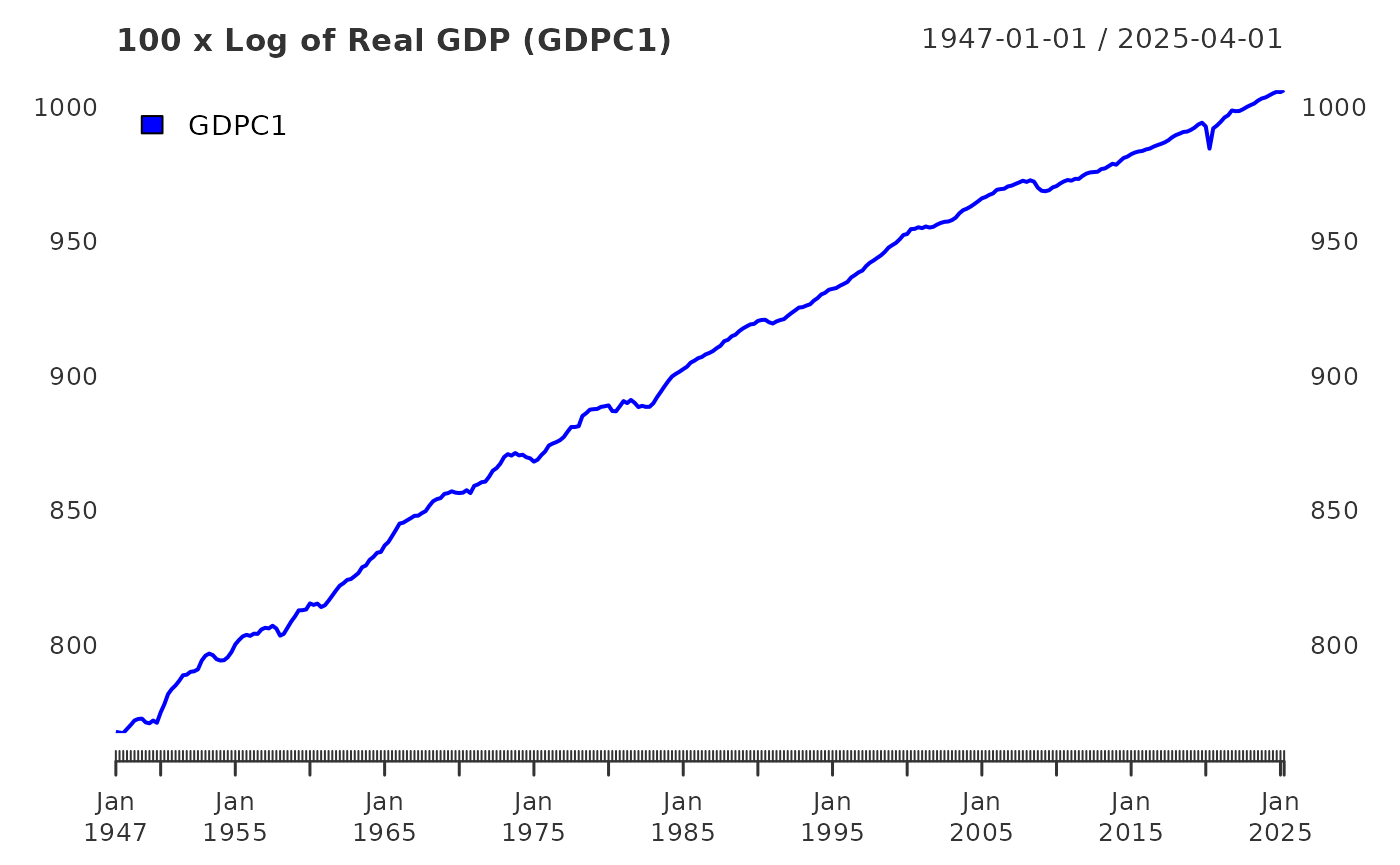addPanel(yth_filter, output=c("cycle"), type="h", on=NA, col="darkred" )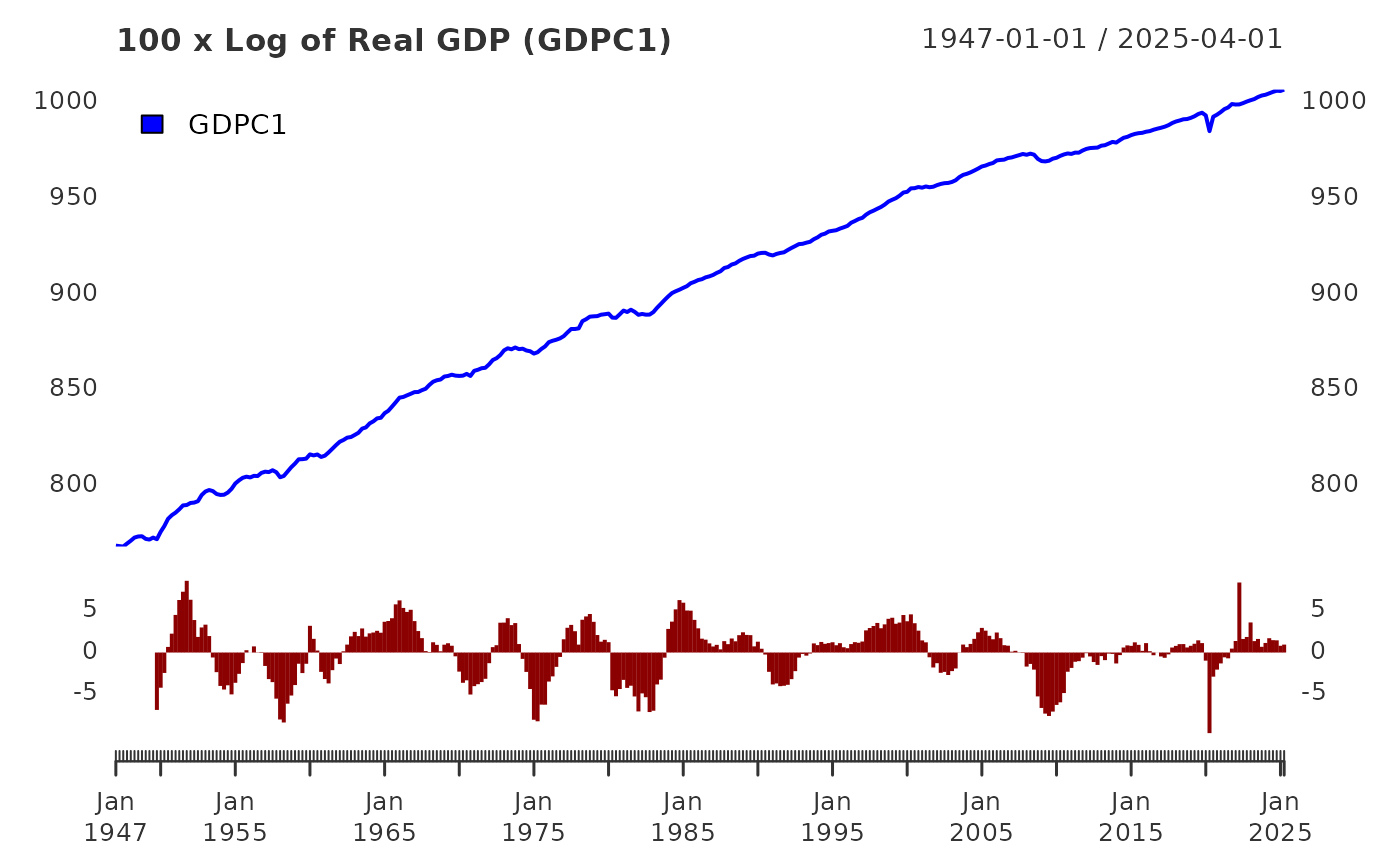## Choices for h and p

In the original paper, Hamilton aggregates the PAYEMS monthly employment series into data of quarterly periodicity prior to apply his filter. That is a desirable approach when comparing with other economic series of quarterly periodicity. However, using the yth_filter function, one can choose to retain the monthly series and adjust the h and p parameters accordingly.

The default parameters of h = 8 and p = 4 assume times series data of a quarterly periodicity. For time series of monthly periodicity, one can retain the same look-ahead and lag periods with h = 24 and p = 12. See the yth_filter documentation for more details.

Employment_log <- 100*log(PAYEMS["1950/"])

employment_cycle <- yth_filter(Employment_log, h = 24, p = 12, output = "cycle")

plot(employment_cycle, grid.col = "white", type = "h", up.col = "darkgreen", dn.col = "darkred",
main = "Log of Employment cycle")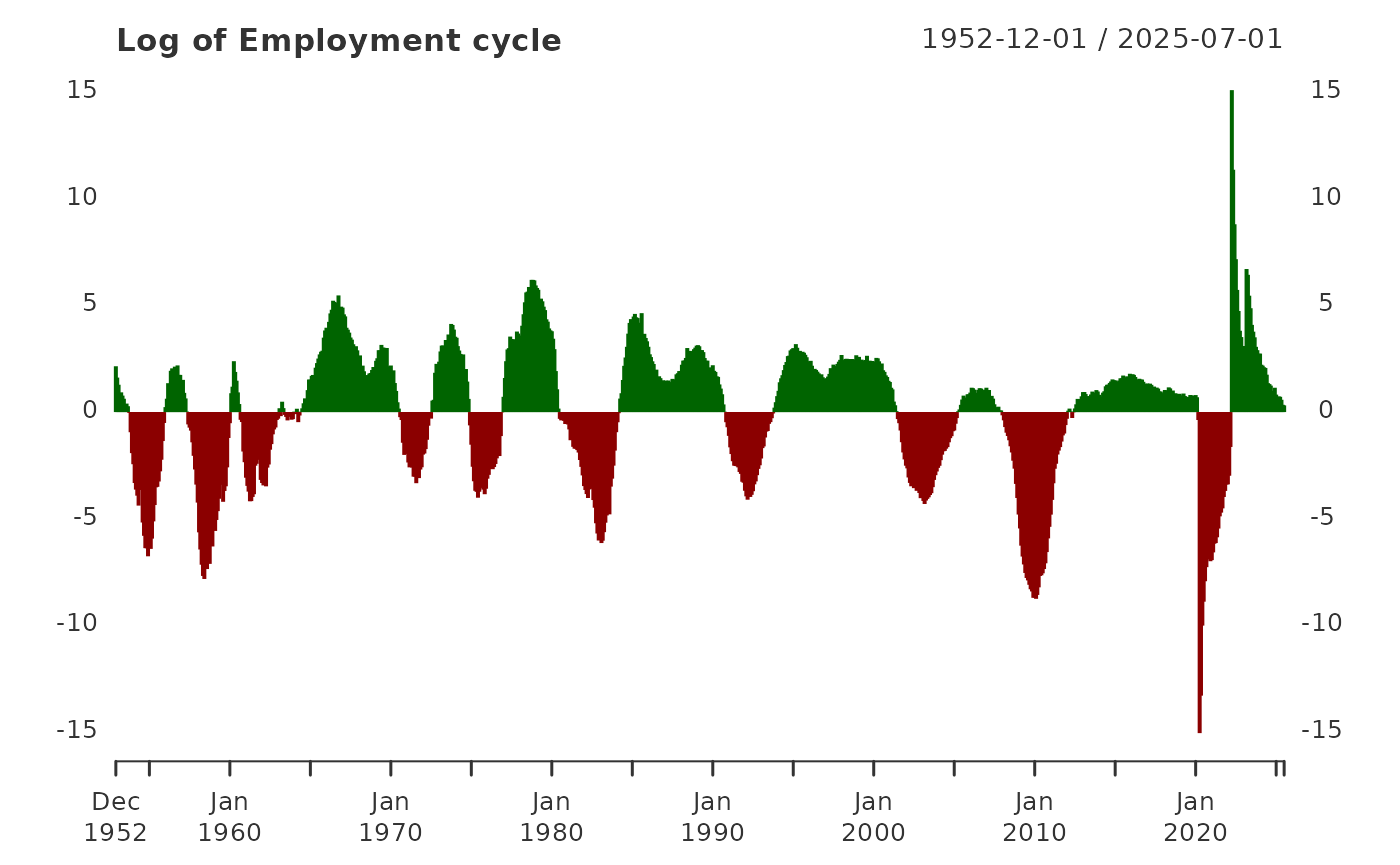In addition to adjusting parameters to accommodate other periodicities, one may wish to explore longer term cycles by extending h. Below are examples of moving the look-ahead period defined by h from 8 quarters (2 years), to 20 quarters (5 years), and then 40 quarters (10 years).

gdp_5yr <- yth_filter(log_RGDP, h = 20, p = 4, output = c("x", "trend", "cycle"))

plot(gdp_5yr["1980/"][,1:2], grid.col = "white", legend.loc = "topleft",
main = "Log of Real GDP and 5-year trend",
panels = 'lines(gdp_5yr["1980/"][,3], type="h", on=NA)')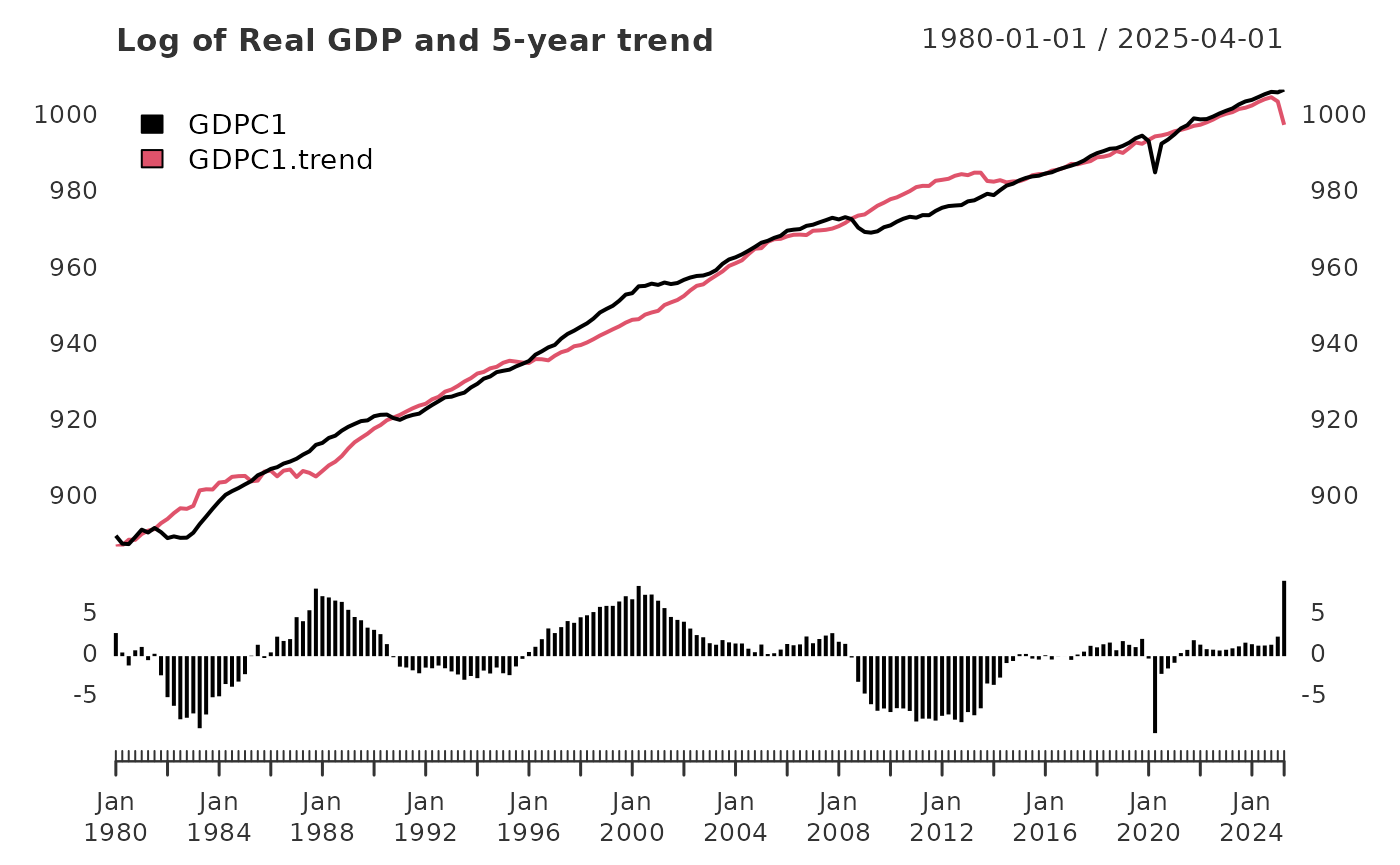gdp_10yr <- yth_filter(log_RGDP, h = 40, p = 4, output = c("x", "trend", "cycle"))

plot(gdp_10yr["1980/"][,1:2], grid.col = "white", legend.loc = "topleft",
main = "Log of Real GDP and 10-year trend",
panels = 'lines(gdp_10yr["1980/"][,3], type="h", on=NA)')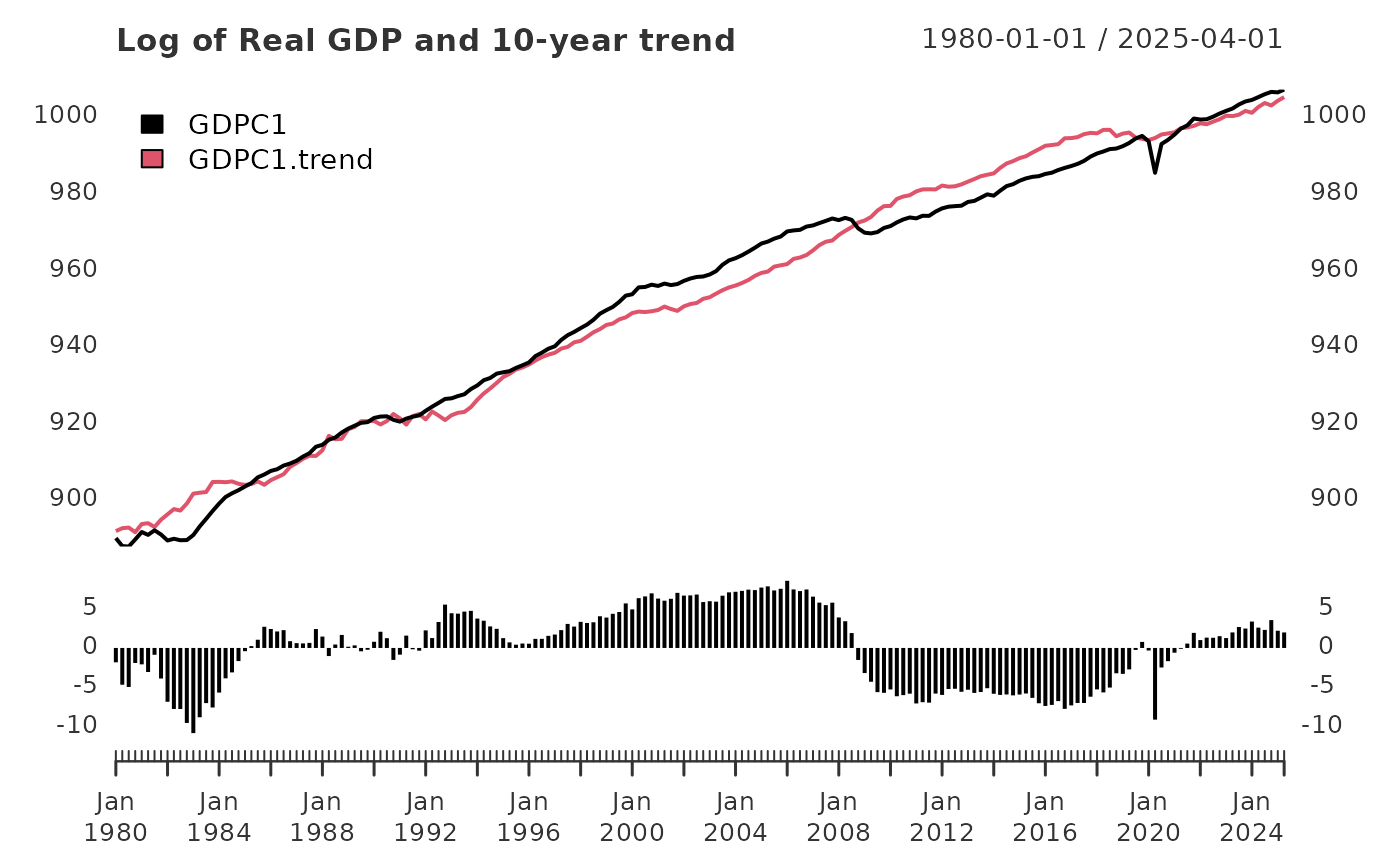## Conclusion

These functions give you an avenue to filter econometric time series into trend and cycle components. They identify a more stable trend and cycle components that doesn’t have the estimation issues associated with the head and tail portions of those generated by the HP-filter.